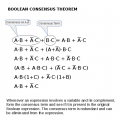# Boolean Algebra: What's the simplest way to prove this?

#### amazun11

Joined Jun 22, 2017
1
Hi everyone. I'm working on practice questions, and I'm supposed to algebraically prove this:

X'Y' + Y'Z + XZ + XY + YZ' = X'Y' + XZ + YZ'

Upon first glance, the terms that aren't in both sides are Y'Z and XY. So, is there an easy way to prove that these two terms are equal to 0, because then LS=RS? I don't know how to prove it the long way, either..

#### RBR1317

Joined Nov 13, 2010
706
So, is there an easy way to prove that these two terms are equal to 0,
Terms will not be equal to zero; however, some terms will be included within combinations of other terms. So the general procedure would be to expand terms on one side, eliminate any duplicate terms, then recombine terms in a way to create the other side.

For instance, X'Y' = X'Y'(Z+Z') = X'Y'Z+X'Y'Z' and XY'Z+XY'Z = XY'Z

#### WBahn

Joined Mar 31, 2012
27,854
Hi everyone. I'm working on practice questions, and I'm supposed to algebraically prove this:

X'Y' + Y'Z + XZ + XY + YZ' = X'Y' + XZ + YZ'

Upon first glance, the terms that aren't in both sides are Y'Z and XY. So, is there an easy way to prove that these two terms are equal to 0, because then LS=RS? I don't know how to prove it the long way, either..
If the two sides are equal, then that means that the term XY has no effect. So see if you can determine WHY that term has no effect. Assume that XY = TRUE. That means that the left side is TRUE, regardless of any other terms on that side and regardless of the value of Z. It also means that the right side must also be TRUE, regardless of Z. Is it? If so, why? That will let you identify the terms on the left that, between them, are able to absorb XY.

#### shteii01

Joined Feb 19, 2010
4,644
There 6 or 8 boolean algebra rules, you apply them one by one until the equation is simplified and none of them can be applied.

#### MrAl

Joined Jun 17, 2014
9,622
Hi everyone. I'm working on practice questions, and I'm supposed to algebraically prove this:

X'Y' + Y'Z + XZ + XY + YZ' = X'Y' + XZ + YZ'

Upon first glance, the terms that aren't in both sides are Y'Z and XY. So, is there an easy way to prove that these two terms are equal to 0, because then LS=RS? I don't know how to prove it the long way, either..
Hi,

Quick question:
Are you supposed to prove that this is true always or that this is conditionally true?

#### WBahn

Joined Mar 31, 2012
27,854
Hi,

Quick question:
Are you supposed to prove that this is true always or that this is conditionally true?
What kind of conditions are you thinking of? Both of the extra terms on the left can be removed using Boolean algebra identities.

#### MrAl

Joined Jun 17, 2014
9,622
Hi,

I did not look into this in detail yet, but i'll have to wait until the OP gets the solution before i say too much more as i dont want to give anything away or mislead the OP. If the problem had a non conditional solution that's fine#### RBR1317

Joined Nov 13, 2010
706
What's the simplest way to prove this?
"Simple" can have different contexts.

Expansion of terms in the Boolean expression to a basic canonical form, elimination of duplicate terms, and recombination of terms is conceptually simple; equivalent to sorting through your grocery list. Just keep track of all the items.

Application of the Boolean Consensus Theorem is not as conceptually simple, but in practice involves merely identifying the consensus terms and eliminating them. For full credit, one would probably need to identify how the consensus term(s) originated.Last edited by a moderator: